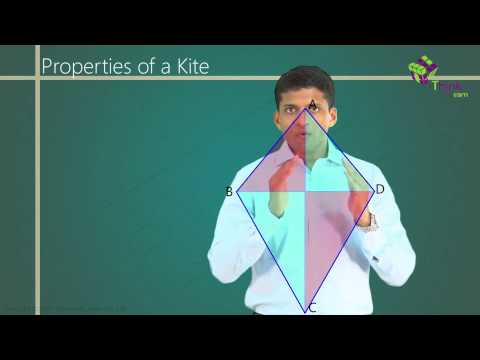A polygon is a plane figure which is bounded by finite line segments to form a closed figure. A 4-sided polygon is known as a quadrilateral.

The sum of interior angles of a quadrilateral is always 360°. The number of diagonals in a n-sided polygon is given by

$$\begin{array}{l} \frac {n(n-3)}{2} \end{array}$$
. Thus, a quadrilateral has 2 diagonals. Also, for any n-sided polygon, the sum of exterior angles of any polygon is always 360°.

Kite is a quadrilateral in which two pairs of adjacent sides are of equal length and the diagonals intersect each other at right angles. The figure shown below represents a kite.

The fig 1 above represents a quadrilateral and it can be represented as:

In quadrilateral ABCD, the sides AB = BC and AD = CD. Thus, as the adjacent pair of sides is equal in length, therefore ABCD is a special type of quadrilateral known as a kite.

Kite Properties:

• i)  Diagonals intersect at right angles.

In a kite, the diagonals intersect at right angles. This can be proved as follows.

From fig. 2 above, in ∆ABD and ∆BCD

AB=BC

BD is common.

Therefore ∆ABD ≅ ∆BCD (SSS rule of congruency)

AB = BC and AD = CD

Thus, ∆ABC is an isosceles triangle.

⇒ ∠BAO = ∠BCO

Thus, ∆ABO ≅ ∆BCO (ASA rule of congruency)

⇒∠AOB = ∠BOC (CPCT)

Also, ∠AOB + ∠BOC = 180° (Linear pair)

⇒ ∠AOB = ∠BOC = 90°

(NOTE: CPCT stands for congruent parts of congruent triangle)

•  ii)  One of the diagonals is the perpendicular bisector of another.

As seen above ∆ABO ≅ ∆BCO and ∠AOB = ∠BOC = 90°

⇒ AO = OC (CPCT)

Thus BD bisects AC at right angles.

• iii)  Angles between unequal sides are equal.

• iv)  Area of a kite:

If length of both diagonals is given as p and q as shown in the figure given below, area (A) of kite can be given as:

A =

$$\begin{array}{l} \frac {p \times q}{2} \end{array}$$

• v) Rhombus as a special case of kite:

If all the sides of a kite become equal in length then that kite becomes a rhombus as sides are of equal length and diagonals are perpendicular to each other.